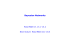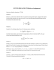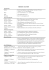# Bayesian Sample Size Determination in Two

## Transcription

Bayesian Sample Size Determination in Two
```Biometrics & Biostatistics International Journal
Bayesian Sample Size Determination in Two-Sample
Poisson Models
Mini Review
Abstract
Sample size determination is a vital part of clinical studies where cost and safety
concerns lead to greater importance of not using more subjects and resources
than are required. The Bayesian approach to sample size determination has
the advantages of being able to use prior data and expert opinion to possibly
reduce the total sample size while also acknowledging all uncertainty at the
design stage. We apply a Bayesian decision theoretic approach to the problem
of comparing two Poisson rates and find the required sample size to obtain a
desired power while controlling the Type I error rate.
Keywords: Bayes factor; Poisson rate; power; Type I error
Introduction
Sample size determination continues to be an important
research area of statistics. Perhaps nowhere is this truer than
pharmaceutical statistics, where cost and time constraints have
made finding the appropriate sample size before conducting a
study of the utmost importance. The problem is quite simple:
too small a sample can lead to under-powered studies, while too
consider the sample size determination problem as it pertains
to the two-sample testing of Poisson rates from a Bayesian
perspective subject to operating characteristics constraints.
There are several advantages to the Bayesian perspective
when trying to determine a study’s requisite sample size, a
topic that is expounded in Adcock . Their construction does
not depend on asymptotic approximations or bounds. Classical
solutions to the sample size determination problem typically
hinge on asymptotic arguments that require the researcher
to specify one parameter value (perhaps vector valued) as
representative for the entire parameter space, a process that
is typically done using bounding arguments. This, for example,
is what is done when determining the requisite sample size
for a confidence interval of a fixed level and given length. The
resulting sample sizes are consequently conservative. On the
other hand, the Bayesian approach provides the statistician with
the ability to model his indecision about the parameter through
expert knowledge or previous studies. As noted by Bayarri and
Berger , this can allow the Bayesian approach to have better
operating characteristics, such as a smaller required sample
sizes or better Type I and II error rates.
Various Bayesian sample size determination methods
have been studied for binomial and Poisson data. Stamey et
al.  considered one and two sample Poisson rates from the
perspective of interval based criteria such as coverage and width.
Hand et al.  extend those ideas by considering both intervalbased and test-based criteria, albeit without considering power.
Katsis and Toman  used more decision theoretic criteria
for the two sample binomial case, but only to the extent of
controlling the posterior risk with a pre specified bound. Zhao
Submit Manuscript | http://medcraveonline.com
Volume 2 Issue 1 - 2015
Ryan Sides, David Kahle* and James
Stamey
Statistical Science, Baylor University, USA
*Corresponding author: David Kahle, Department of
Statistical Science, Baylor University, Waco, TX, USA, Tel:
254-710-6102; E-mail:
Received: February 10, 2015 | Published: March 09, 2015
et al.  extend those ideas by using computational methods to
extend these results to the Poisson data model. We also consider
the problem the subject to Type I and Type II constraints. This is
thus an important extension of , because it
1) Extends the ideas to the Poisson case
2) Enables the incorporation of operating characteristics.
A subtle difference between the classical and Bayesian
methods of sample size determination merits discussion before
algorithmic solution to the sample size determination problem
subject to operating characteristics constraints for Poisson data.
However, since the entire problem is treated in a Bayesian context,
the concept of Type I and Type II error rates is understood in an
average, or expected, sense; see . For example, the ``power of
a test’’ retains the interpretation of the probability the decision
rule rejects when the null hypothesis when it is false; but rather
than being a function defined over the alternative space, here it is
averaged over that space and weighted by the prior distribution
specified on the alternative hypothesis. To make the distinction
more clear, we refer to this as the expected Bayesian power
(EBP), as is done in ; alternatively, it may be referred to as the
probability of a successful test. These ideas, though apparently
not considered in the literature previously, can also apply to
the concept of the significance level of a test. While frequentist
methods typically report one value for significance level, what
they are really doing (in non point null hypotheses) is taking the
largest possible significance level; thus, taking an expectation of
a significance level curve could be done as well. Consequently, in
level (EBSL), defined as the expected value of the test under the
prior distribution given on the null space. In both cases, any
particular instance of the actual Type I and Type II error rates
can be greater than or less than nominal.
the theoretical formulation of the sample size determination
problem for two Poisson variates including consideration of
Biom Biostat Int J 2015, 2(1): 00023
Bayesian Sample Size Determination in Two-Sample Poisson Models
operating characteristics. In Section 3, we present an algorithmic
solution to the sample size determination problem posed in
Section 4. Section 5 contains an application of the method in
the area of pharmaceutical statistics. We then conclude with a
discussion.
Problem specification and the bayes rule
We now follow the general framework of  in the
development of this problem, adapting the binomial case
to fit the Poisson data model. Suppose Y1 ~ Pois (tλ1 ) and
Y2 ~ Pois (tλ 2 ) , independently, where λ1 and λ 2 represent the
rate parameters of interest and t represents a common sample
(or “opportunity”) size. Together, we write these Y = (Y1 , Y2 )′
with observations y = ( y1 , y2 )′ . The sample size determination
problem is to calculate the necessary sample size required to
test the hypotheses H 0 : λ1 = λ2 vs H1 : λ1 ≠ λ2 2/5
expected loss . Since the posterior expected loss associated
with an action a ∈ {0,1} is
ρ(a=
) Eλ | y [ L(ϕ, a )]
( H 0 | Y y=
), if a 1,
 c P=
= 1
Y
y
c
P
=
(
H
|
=
),
if a 0,
1
 2
Setting c = c1 / c2 we can express the optimal decision
rule, a* , as
y ) < cP( H 0 | Y =
y ),
0, if P( H1 | Y =
a* ( y ) = 
y ) ≥ cP( H 0 | Y =
y ).
1, if P( H1 | Y =
The rejection region, W , is therefore
W=
{ y : P ( H1 | Y =
y ) ≥ cP( H 0 | Y =
y )}.
The optimal rule in (2) can be nicely represented in
terms of the Bayes factor. The Bayes factor is defined as the ratio
of the posterior odds of H1 to the prior odds of H1 , so that a large
Bayes factor is evidence for rejecting the null hypothesis .
Specifically, the Bayes factor is defined
using a given decision rule; here we use the Bayes rule.
Denoting the parameter pair λ = (λ1 , λ 2 )′ , the associated null
and alternative spaces are therefore
which
P ( H1 | Y= y ) / P ( H 0 | Y= y ) P ( H1 | Y= y )π0
=
B =
.
λ , and
we identify with R+ with elements generically denoted
π1 / π0
P ( H 0 | Y = y )π1
.
This ratio is useful in Bayesian inference because it is often
interpreted as partially eliminating the influence of the prior on
As this problem is being considered from the Bayesian
the posterior, instead emphasizing the role of the data. Moreover,
perspective, we place prior probabilities of π 0 and π 1 = 1 − π 0
the decision rule is a function of a Bayes factor:
on H 0 and H1 , respectively. Conditional on the null being true,
W=
y ) ≥ cP( H 0 | Y =
y )}
{ y : P ( H1 | Y =
we represent the expert opinion regarding λ as p0 (λ) , defined

π
π 
over the set Λ 0 . Alternatively, conditionally on H1 being true,
=
y ) 0 ≥ cP( H 0 | Y =
y) 0 
 y : P ( H1 | Y =
π
π1 
we represent the belief concerning λ1 ≠ λ 2 with a joint prior
1


π0 
p (λ1 , λ 2 ) with support Λ1 . Marginalizing over the hypotheses,
=  y : B ≥ c .
π1 
we have the unconditional prior

This is particularly useful because it allows for the
p (λ1 , λ 2 )= p0 (λ )π0 I H 0 + p1 (λ1 , λ 2 )π1 I H1 ,
interpretation of the Bayes factor B as the test statistic for the
decision rule in (2); this is the condition in (4).
Where I H 0 and I H1 are the indicator function of H 0 and H1
, respectively.
We now derive closed-form expression for the posterior
probabilities of the null and alternative hypotheses. Using Bayes’
In practice, the prior distributions on λ , λ1 , and λ 2
summarize expert opinion concerning the parameters in each of theorem, we have
the two scenarios H 0 and H1 . This information can be obtained
=
P (Y y | H 0 )π0
P ( H 0 | Y= y=
)
in a number of ways including past data, prior elicitation of
P=
(Y y | H 0 )π0 + P=
(Y y | H1 )π1
expert opinion (see especially ), or based on uninformative
and
criteria. For simplicity, we consider conjugate priors for all
=
P (Y y | H1 )π1
three λ ’s, so that under H 0 , λ ∼ Gamma(α, β) , and under H1
P ( H1 | Y= y=
)
.
P
=
(
Y
y
|
H 0 )π0 + P=
(Y y | H1 )π1
, λ i ∼ Gamma(α i , βi ) independently. This assumption is not
Consequently, the posterior probabilities have closed-form
very restrictive and allows us to specify parameters of prior
expressions
if the likelihoods do. Computing these, we have
distribution sin stead of distributions themselves.
∞
We now derive the optimal Bayes (decision) rule in deciding
between the hypotheses presented in (1). In solving the related
problem between two binomial proportions,  use the classical
decision theoretic setup using the 0-1 loss function . Here
we use the more general unequal loss function
c1 if H 0 is true and a = 1,

L( H , a ) = c2 if H1 is true and a = 0,
 0, otherwise

Where a = δ( y ) is the decision rule with a = 0 representing
selection of H 0 and a = 1 selection of H1 . Thus, c1 represents the
loss associated with a Type I error, and c2 that of a Type II error.
The Bayes action is simply the one that minimizes posterior
∫ f ( y | λ, H ) p (λ | H ) d λ
P(Y = y | H 0 ) =
0
0
0
0
(λt ) y1 e −λt (λt ) y2 e −λt βα α−1 −βλ
λ e dλ
y1 !
y2 ! Γ ( α )
0
∞
∫
=
=
t y1+ y2 βα Γ( y1 + y2 + α)
,
y1 ! y2 !Γ(α)(2t + β) y1+ y2 +α
and
=
P(Y y=
| H1 )
∞
∫ f ( y | λ, H ) p (λ | H ) d λ
1
1
−λit
1
βi
λ i αi −1e −βiλi d λ i
Γ (α i )
0
=
2
∞
∏∫
i =1 0
2
=∏
i =1
(λ i t ) e
yi !
yi
αi
t yi βi αi Γ( yi + α i )
.
yi !Γ(α i )(t + βi ) yi +αi
Citation: Ryan S, David K, James S (2015) Bayesian Sample Size Determination in Two-Sample Poisson Models. Biom Biostat Int J 2(1): 00023. DOI:
10.15406/bbij.2015.02.00023
Bayesian Sample Size Determination in Two-Sample Poisson Models
Note that the probability of the data under the null hypothesis
is the product of two independent negative binomial likelihoods.
Combining (3) with (5) and (6) allows us to represent W in
terms of the null and alternative likelihoods as follows:
W=
y ) ≥ cP ( H 0 | Y =
y )}
{ y : P ( H1 | Y =
=
(Y
{ y : P=
y | H1 )π1 ≥ cP
=
(Y y | H 0 )π0 }.
Consequently, (7) and (8) give explicit conditions for the
rejection of the optimal decision rule:

Γ(α)(2t + β) y1+ y2 +α 2 βi αi Γ( yi + α i )
π 
y:B
W =
=
≥c 0
∏
yi +αi
α
β Γ( y1 + y2 + α) i=1 Γ(α i )(t + βi )
π1 

Note that the left side of (9) is our test statistic and Bayes
factor, B, so that (9) is an explicit formulation of the condition
presented in (4).
Sample Size Determination for the Bayes Rule
The explicit description of the decision rule in (9) allows us
to compute all sorts of quantities of interest. For given prior
parameters π0 , π1 , α, β, α1 , β1 , α 2 , and β2 and loss penalties
c1 and c2 (or simply c), the Expected Bayesian Power (EBP) ωt
is defined
ω
=
P(Y ∈ W | H=
)
t 1
Y
∑ P(=
α=
P (Y ∈ W | H=
)
t
0
∑ P(Y=
y∈W
y | H=
)
1
t yi βi αi Γ( yi + α i )
2
∑∏ y !Γ(α )(t + β )
y∈W i =1
i
i
i
,
yi +αi
and the Expected Bayesian Significance Level (EBSL) α t is
y∈W
y | H=
)
0
t y1+ y2 βα Γ( y1 + y2 + α)
∑ y ! y !Γ(α)(2t + β)
y∈W
1
2
y1 + y2 +α
.
Note three things. First the inclusion of the t subscripts
highlights the fact that these quantities depend on t. Second,
both ωt and α t marginalize over the corresponding alternative
and null spaces Λ1 and Λ 0 , respectively, this is the sense in
which the power and significance level are expectations. Third,
the constant c (or c1 and c2) is represented in the expressions
through Wt, which is itself dependent on t.
In their articles, [5, 12] demonstrate that as the sample size
tends to infinity, the Bayes factor B converges to either 0 or 1. As
a consequence, in the current context as the sample size t tends
to infinity the Bayes factor B converges to either 0 or 1, so that
a.s.
a.s.
→1 and α t 
→ 0 . Thus, for any pre implies that ωt 
ω
specified power and significance level α , there exists a t such
that for all t ′ ≥ t * , ωt′ ≥ ω and α t′ ≥ α . We define tα* ,ω to be the in
fimum of this collection of lower bounds, i.e.
=
tα* ,ω inf
{t : αt ≤ α and ωt ≥ ω} .
t ∈R
+
tα* ,ω is said to be the optimal sample size for ω and α , and
computing t * is called the sample size determination problem.
If only a power is specified, or if only a significance level is
specified, the other quantity is left off of the subscript and out of
the definition. We often write simply t * for tα* ,ω .
Were (10) and (11) monotonic and continuous in t, the sample
size determination problem would be quite straightforward.
3/5
Simply run a numerical root-finder (e.g. Newton’s method)
on ωt − ω and α t − α take the larger t. Unfortunately, however,
as a function of t both the power and significance level
are discontinuous functions that are not monotonic. As a
consequence, it is possible, for example, for there to be two
such sample sizes t1and t2 with t1 < t2 such that ωt1 ≥ ω and yet
ωt2 < ω . This is a consequence of the dependence of Wt on t: as t
grows, the rejection region changes, and these changes result in
discrete jumps in the rejection region.
Practically speaking, in our experience the appearance of
these jumps is monotonically decreasing in magnitude and
dissipate quite quickly in t so that, while there are jumps, they
become relatively minor even for quite small t. Thus, while outof-the-box numerical routines are insufficient for the task, a
straight-forward heuristic algorithm suffices to certify t * to a
reasonable level of accuracy.
Initialize t = t0 with a value small enough to satisfy ωt < ω
and α t > α , typically a value like .1 will suffice. Then, double t
until both conditions are met. Once both conditions are met,
decrement t by 1 until the conditions are no longer satisfied;
then decrement by .1, and so on to achieve the desired precision.
Alternatively, one may move in a binary search manner; this
method is faster but loses the certificate of the solution up to the
highest evaluated point. By contrast, the first heuristic certifies
that the sample size achieved is optimal up to the largest t
observed, which is of the form 2k t0 .
One computational detail is relevant for implementing this
procedure. By definition, since Y1 and Y2 are Poisson variates,
their sample spaces are infinitely large, and thus computing
ωt and αt is not numerically possible - one cannot check every
y to determine whether or not it is included in Wt. There is,
however, a very reasonable work-around for this problem using
prior predictive distributions. Under H1, the prior predictive
distributions on Y1 and Y2 are both negative binomial. Specifically,


β 
β 
Y1 ~ NegBin  α1 , 1  and Y2 ~ NegBin  α 2 , 2  .
t
+
β
t
+
β2 
1 


While one cannot enumerate the entire sample space of Y ,
one can be confident they have a satisfactory approximation by
computing small (e.g. .00001) and large (e.g. .99999) quintiles
of these distributions and then simply taking every combination
of the ranges from low to high. This is the approach we take to
computing the sums listed in (10) and (11) above.
An Example from Cancer Therapeutics
1) An example of the proposed methodology is readily
available from the field of cancer therapeutics. Suppose
(1) Drug A is an industry standard therapy for a certain
type of cancer that is known to have the common side
effect of mild seizures every hour of infusion
2) (2) Drug B is a novel compound believed to have the
same side effect but at a lessened rate
3) (3) The goal is to design a design a clinical trial that
compares the two using the minimum resources
required to meet 5% significance level (EBSL) and
80% power (EBP). Moreover, suppose that the losses
associated with Type I and Type II errors have a ratio
of 1:1.
Citation: Ryan S, David K, James S (2015) Bayesian Sample Size Determination in Two-Sample Poisson Models. Biom Biostat Int J 2(1): 00023. DOI:
10.15406/bbij.2015.02.00023
Bayesian Sample Size Determination in Two-Sample Poisson Models
4/5
From past studies, it is known that the uncertainty in the
rate of seizures with Drug A (per hour) is well-represented by
a Gamma (4, 4) distribution so that λ A ~ Gamma(4, 4) . Drug B, by
contrast, is believed to be a bit worse, with perhaps a rate that
is double that of Drug A with experts 90% sure the value is less
than about 3. This translates into roughly λ B ~ Gamma(4,8) . Figure
1 shows both of these priors graphically.
Figure 3: EBSL curve
Figure 1: Prior structures used in Poisson sample size determination example.
Assuming that the rates are the same, the past indicators of
Drug A supersede the lack of evidence for Drug B, so that under
the null hypothesis λ = λ1 = λ 2 ~ Gamma(4, 4) is most appropriate.
Assuming that the null and alternative hypotheses are given the
same belief (50%), the rejection rule from (9) is therefore
W
 Γ(4 )(2t + 4) y1+ y2 +4 4 4 Γ( y1 + 4) 48 Γ( y2 + 8)

≥ 3
y: 4
4 Γ( y1 + y2 + 4) Γ(4)(t + 4) y1+4 Γ(8)(t + 4) y1+8


 (2t + 4) y1+ y2 +4 15120 ( y1 + y2 + 3)! 
= y:
≥

(t + 4) y1+4
65536 ( y1 + 3)!( y2 + 7)!

To design a test with 80% power and at the 5% significance
level, we need but to run the algorithm described in Section
3. To illustrate the scenario, we plot the significance level and
power for every t from 1 to 80; these are included in Figures 2
and 3, respectively. Note how quickly (in t) the functions become
smooth, nullifying any concern about jumps. The 80% level is
achieved at t = 37, with a power of 80.1%, and the 5% significance
level is achieved at t = 57, where the significance level is 4.9%.
Thus, to achieve both, we select the higher sample size, t = 57.
Figure 2: EBP curve
We can also verify these results via simulation by generating
one million random values of λ1 and λ 2 from the priors given
above. To validate the significance level result, we simply (1)
sample from the prior, (2) generate two Poisson observations
with mean equal to the sample in (1) times 57, and (3)
determine whether the test rejects (1) or not (0). Averaging the
million test results, the value is confirmed to Monte Carlo error
(code available upon request). To validate the power result, we
(1) sample one number from a Gamma(4, 4)and one number
from a Gamma(8, 4), (2) sample two Poisson observations from
distributions with mean 37 times the two variates generated in
(1), and (3) determine whether the test rejects or not. Averaging
the million results validates the theoretical result.
Discussion
In this paper we have used conjugate prior structures in
order to assess our beliefs about a rate parameter in a two
sample Poisson trial a priori in order to find the minimal sample
size needed to reach certain operating characteristics. By the
use of a loss function constant, we are able to control for either
the desired expected Bayesian significance level or the desired
expected Bayesian power or both. This type of analysis had
not been considered previously, specifically, simultaneously
controlling for both Type I and Type II error.
Future work includes the consideration of analysis priors in
this research. Ideally, we would be able to adapt this research to
account for the fact that researchers often times use one set of
priors when conducting sample size analyses, but a more vague
or non-informative set of priors when actually analyzing the
experiment. Though we used only mildly informative priors in
our example, it may be the case where a substantially informative
prior is required for the design stage but a less informative prior
would be used in the analysis stage. Further, this process could
be expanded to consider non-conjugate priors as well. However,
the analytical tractability of conjugate priors made the Poisson/
gamma model ideal, and modeling prior beliefs of a Poisson rate
with a gamma distribution is not an unreasonable thing to do.
It also should be noted that time considerations, while
already improved throughout the process, can always continue
to improve. One improvement to current methods involves
replacing the bi-sectional approaches with one that approximates
the EBP and EBSL curves with some logarithmic function;
Citation: Ryan S, David K, James S (2015) Bayesian Sample Size Determination in Two-Sample Poisson Models. Biom Biostat Int J 2(1): 00023. DOI:
10.15406/bbij.2015.02.00023
Bayesian Sample Size Determination in Two-Sample Poisson Models
this improvement should get us in the ballpark of a candidate
solution much quicker. Future work also includes a more in
depth look at how expected Bayesian error rates compare to
typical frequentist ones, and potentially an in-depth look at
the different sample size determination and testing methods in
each. Further, we could generalize the algorithm such that we
are not looking at a common sample size t= t1= t2 , but rather
two different sample sizes t1 and t2 such that they do not need to
be equal. Lastly, code is available upon request.
References
1. Adcock CJ (1997) Sample Size Determination: A Review. The
Statistician 46(2): 261-283.
2. Bayarri MJ, Berger JO (2004) The Interplay of Bayesian and
Frequentist Analysis. Statistical Science 19(1): 58-80.
3. Stamey JD, Young DM, Bratcher TL (2006) Bayesian Sample-Size
Determination for One and Two Poisson Rate Parameters with
Applications to Quality Control. Journal of Applied Statistics 33(6):
583-594.
4. Hand A, Stamey JD, Young, DM (2011) Bayesian Sample-Size
Determination for Two Independent Poisson Rates. Computer
5/5
Methods and Programs in Biomedicine 104(2): 271-277.
5. Katsis A, Toman B (1999) Bayesian Sample Size Calculations for
Binomial Experiments. Journal of Statistical Planning and Inference
81(2): 349-362.
6. Zhao Z, Tang N, Li Y (2011) Sample-Size Determination for Two
Independent Binomial Experiments. Journal of Systems Science
and Complexity 24(5): 981-990.
7. O’Hagan A, Stevens J, Campbell M (2005) Assurance in Clinical
Design. Pharmaceutical Statistics 4(3): 187-201.
8. Speighelhalter DJ, Abrams K R, Myles J P (2004) Bayesian
Approaches to Clinical Trials and Health Care Evaluation. Wiley.
9. Garthwaite PH, Kadane JB, O’Hagan A (2005) Statistical methods
for eliciting probability distributions. Journal of the American
Statistical Association 100: 680-701.
10. Shao J (2003) Mathematical statistics. Springer (2nd edn) pp. 592.
11. Berger J (1985) Statistical decision theory and Bayesian analysis.
Springer.
12. Kass R, Raftery A (1995) Bayes Factors. Journal of the American
Statistical Association 90(430): 773-795.
Citation: Ryan S, David K, James S (2015) Bayesian Sample Size Determination in Two-Sample Poisson Models. Biom Biostat Int J 2(1): 00023. DOI:
10.15406/bbij.2015.02.00023
```

### Infinite Edge ParOOon Models for Overlapping Community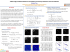### Document 6590118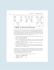### Guide to Benefits Analysis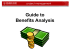### Exercise: 8 Q1. Let X and X be independent random variables, X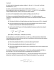### 2015 Provider Contact Information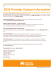### Singer AND lover were executed then made to look### A Statistical Quality Management Framework for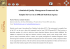### Bayesian Networks